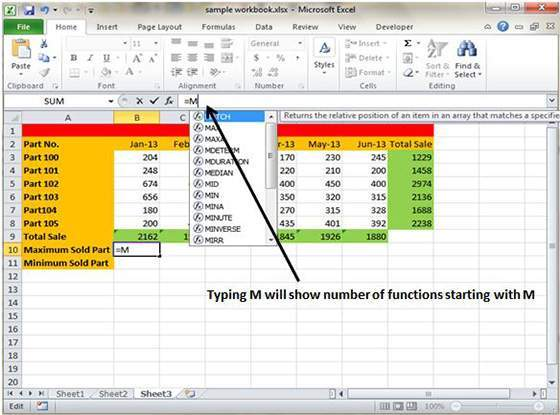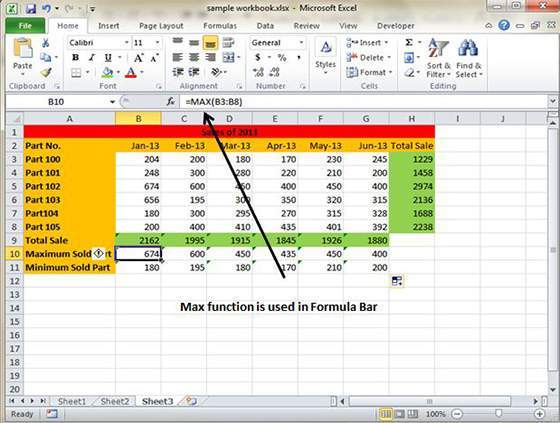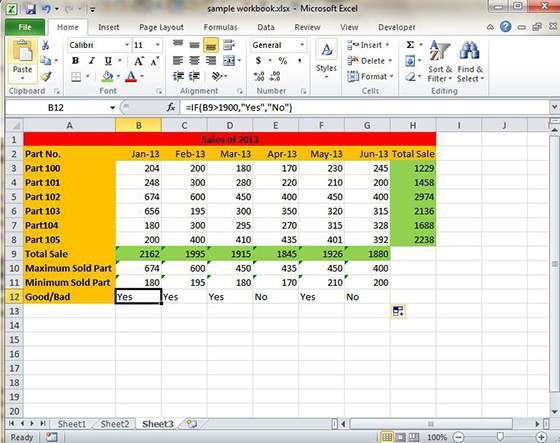# How To Use Functions In MS Excel 2010

A large number of formulas make an active use of the functions that are present in the worksheet. These functions will enhance the power of the formulas and makes them perform some of the complex calculation with ease and simplicity.

For example, you can make use of the LOG or SIN function in order to calculate the Logarithm or Sin ratio. It is not possible to perform the complicated and complex calculation using the mathematical operators and functions comes to the rescue in such scenario.

Using Functions

Whenever you type = sign followed by an alphabet, it will show a large number of functions to choose from.Let us assume that you are required to determine the largest value in the range. A formula won’t be able to deliver the result without making use of a function. Herein, we will supply the MAX function to the formula, which will help in returning the largest value in the range of B3:B8. The formula will be =MAX(A1:D100)Another example will help in understanding the use of functions in formulas better.  Assume you are required to find if the cell of the month is greater than 1900, so that you can give Bonus to the sales representative. This can be done by simply passing the IF function in the formula. The formula will be like this =IF(B9>1900,”Yes”,”No”).Function Arguments

You must have noticed that all the functions that have been used in the above example makes uses of parentheses. The information placed inside the parentheses is the list of arguments.

All functions vary in the way they make use of these arguments. Depending on what the function has to perform, a function makes a careful use of the arguments.

• No arguments– It examples are Now(), Date() etc.
• One argument– UPPER(), LOWER(), ETC.
• A fixed number of arguments: IF(), MAX(), MIN(), AVERAGE(), etc.
• Infinite number of arguments
• Optional arguments